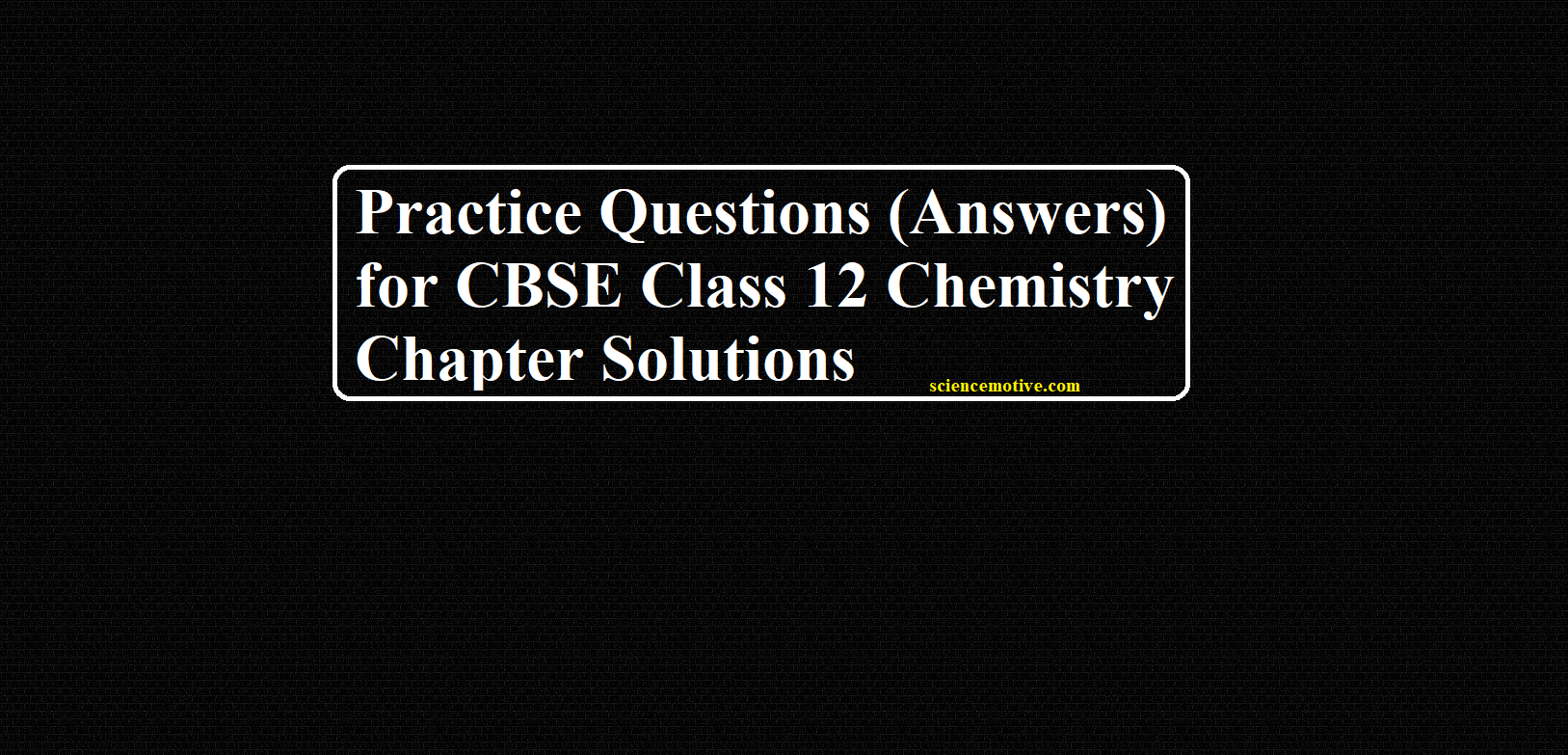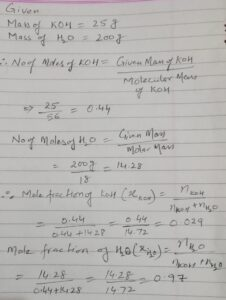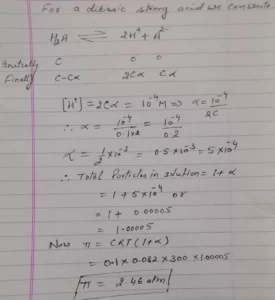# Practice Questions (Answers) for CBSE Class 12 Chemistry Chapter Solutions# Practice Questions (Answers) for CBSE Class 12 Chemistry Chapter Solutions

Class 12 Chemistry Chapter Solutions

Ans 1. Non-Volatile

Ans 2. Negative

Ans 3. Positive

Ans 4. The dissolution of a gas in a liquid is an exothermic process. Therefore, according to the Le-Chatelier principle, with the increase in temperature, the equilibrium shifts in the backward direction.
Gas + Liquid  →  Solution + Heat
Therefore, the solubility of a gas in solution decreases with rising temperature.

Ans 5. Given, W = 25gAns 6. The solution has a negative deviation from Raoult’s law because the decrease in volume indicates strong forces of interaction between the molecules in the solution.

Ans 7. Since the dissolution of NH4Cl in water is an endothermic process, its solubility increases with rising in temperature.

Ans 8. The Vapour pressure of an aqueous solution of glucose lower than that of water, it is because in glucose, the surface of liquid consists of both solute and solvent molecules, therefore, the fraction of surface covered by the solvent molecules gets reduced.
Hence, escaping tendency of solvent molecules into vapors also gets reduced, thus, vapor pressure of glucose solution decreases.

Ans 9. PN2 = XN2 × KH
KH = 105 atm
Mole fraction of N2 = 0.8
∴ Partial pressure of N2 = 0.8 × 5 = 4
XN2 × 105 = 4
∴ XN2 = 4 × 10-5
Number of moles of H2O = 1 mole
Number of Moles of N2 = ?
XN2 = No. of moles of N2 / No. of moles of N2 + No. of moles of H2O
4 × 10-5 = No. of moles of N2 / No. of moles of N2 + No. of moles of H2O
XN2 = 4 × 10-5

Ans 10. The egg placed in water will swell due to the osmosis of pure water into the egg. On the other hand, the egg placed in the saturated solution of NaCl will shrink due to the osmosis of water out of the egg. This is because osmosis always occurs from a higher concentration of solvent to a lower concentration of solvent.

Class 12 Chemistry Chapter Solutions

Ans 11.  Liquids having similar structures and polarities form an ideal solution.

Ans 12.  When ethylene glycol is added to a car it reduces the freezing point of water. It is used as an antifreeze in car radiators during winters.

Ans 13. K2SO4 → 2K+ + SO42−
K2SO4 is completely dissociated so
i = 2 + 1 = 3

Ans 14.Ans 15. let the mass of water(solvent) be 1 kg
number of moles of NaCl (nNaCl​ )  = 1 mole
number of moles of urea (nurea )      = 1 mole
molality =  number of moles of solute/ mass of solvent (in kg)
molality ( m​NaCl ) = nNaCl / mass of water  =  1 molal
molality (nurea )    = nurea  /  mass of water  =  1molal
Elevation in boiling point ∆Tb = Tb – Tb = kb × molality
In case of urea
∆T=  Tb – 373.15  =  0.52 x 1
Tb = 0.52 + 373.15 = 373.67 K
Depression in freezing point: ∆Tf​ = Tf – Tf​​  =  k× molality
In case of NaCl
∆Tf = 273.15  – T =  1.86 x 1
T=   273.15  –  1.86 = 271.29K
ratio of ​Tb vs T  =  ​373.67/271.29 =  1.377

Class 12 Chemistry Chapter Solutions

Ans 16. A process by which molecules of a solvent tend to pass through a semipermeable membrane from a less concentrated solution into a more concentrated one.
If a cell is placed in an isotonic solution, there will be no net flow of water into or out of the cell, and the cell’s volume will remain stable. If the solute concentration outside the cell is the same as inside the cell, and the solutes cannot cross the membrane, then that solution is isotonic to the cell.

Ans 17. At 373K V.P of dilute solution of urea is 740 mm Hg
We know that, (P0-P) / P0 = w × M / m × W
Molality = (P0-P) / P0 ×1 000/M
= (760 – 740)/760 × 1000/60 = 0.43 m
Mole Fraction = (P0-P) / P0 = (760-740)/760
= 20/760 = 0.02

Ans 18. 0.25 molal aqueous solution of urea represents 0.25 mole of urea are present in 1 kg of water
Moles of urea = 0.25mole
Mass of solvent (water) = 1 kg = 1000 g
Molar mass of urea (NH2​CONH2​) = 60 g mol
0.25 mole of urea = 0.25 mol × 60 g mol = 15g
Total mass of solution = 1000 + 15 g = 1015 g = 1.015 kg
1.015 kg of solution contain urea = 15 g × 2.5 kg of solution will require urea
= 15g × 2.5 kg / 1.015 kg
= 37 g

Ans 19. As given in the question, a solution of ethylene glycol and water contains 30% of ethylene glycol by mass.
Therefore, in 100g of solution 30g of ethylene glycol and 70g of water is present.
Molar mass of ethylene glycol – (12 × 2 + 1 × 6 + 16 × 2) = 62 g/mol.
So, in 30g we have 30/62 = 0.48 mol.
Number of moles of water = 70/18 = 3.89 mol
Mole fraction of ethylene glycol = No of moles of ethylene glycol/ Total number of moles.
= 0.48 / (0.48 + 3.89) = 0.48 / 4.37 = 0.11
Mole fraction of water = 1 – 0.11 = 0.89

Class 12 Chemistry Chapter Solutions

To Get Question Click the Link Below

Practice Questions for CBSE Class 12 Chemistry Chapter 2